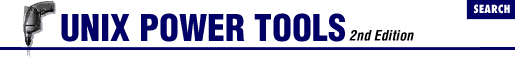home | O'Reilly's CD bookshelfs | FreeBSD | Linux | Cisco | Cisco Exam

#Chapter 49Working with Numbers## 49.2 bc: Hexadecimal or Binary Conversion

One thing that's really handy to know about bc is how to use it for base conversion.

By default, bc takes its input and prints its output in decimal. However, you can set either the input or the output to be some other base numbering system - for example, hexadecimal or binary - using the ibase and obase commands.

For example, to find the decimal equivalents to a hexadecimal number, set ibase to 16, and leave obase alone (i.e., as decimal). Simply type the number (or a series of numbers separated by semicolons) you want converted, and press RETURN. The decimal equivalent will be printed below. (Hexadecimal numbers from A to F must be typed in uppercase, or bc will report an error.) For example:

```% ```bc
ibase=16
B6;7F;FFF
```

182
127
4095```

Or if you wanted to convert to hexadecimal, you'd set obase to 16, and leave ibase at 10:

```% ```bc
obase=16
142
```

8E```

Or, to convert binary to hexadecimal, set `ibase=2` and `obase=16` (or `ibase=16` and `obase=2` for the reverse operation):

```% ```bc
obase=16
ibase=2
11010001
```

D1```

Type CTRL-d to exit bc . Be careful to set obase before ibase , or you will have problems (49.3 ) .

- TOR49.1 bc: Simple Math at the Shell Prompt49.3 Gotchas in Base Conversion